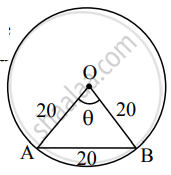# Answer the following : In a circle of diameter 40 cm, the length of a chord is 20 cm. Find the length of minor arc of the chord. - Mathematics and Statistics

Sum

In a circle of diameter 40 cm, the length of a chord is 20 cm. Find the length of minor arc of the chord.

#### SolutionLet ‘O’ be the centre of the circle and AB be the chord of the circle.

Here, d = 40 cm

∴ r = 40/2 = 20cm

Since OA = OB = AB,

ΔOAB is an equilateral triangle.

∴ The angle subtended at the centre by the minor

arc AOB is θ = 60° = (60 xx pi/180)^"c"

= (pi/3)^"c"

∴ l (minor arc of chord AB) = rθ

= 2θ xx pi/3

= (20pi)/3"cm".

Concept: Length of an Arc of a Circle
Is there an error in this question or solution?

#### APPEARS IN

Balbharati Mathematics and Statistics 1 (Arts and Science) 11th Standard Maharashtra State Board
Chapter 1 Angle and its Measurement
Miscellaneous Exercise 1 | Q 2. II (10) | Page 13